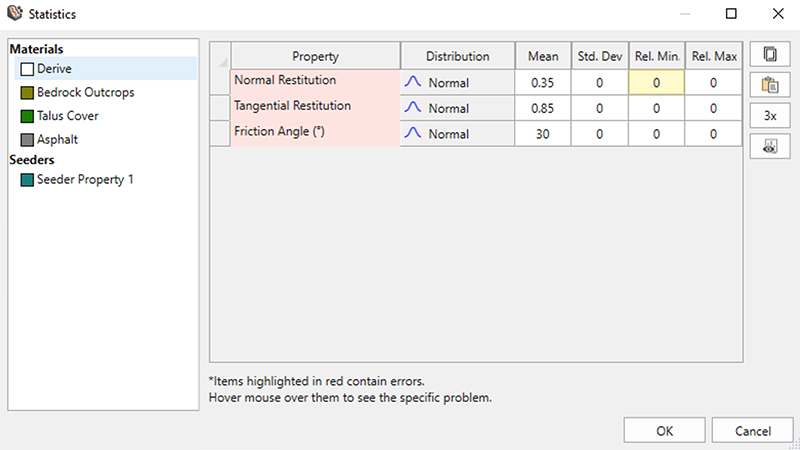Join one of our upcoming courses taking place around the world! Explore now

# Define All Statistics

Each random variable that you define in RocFall3 must have a Statistical Distribution selected. The following statistical distributions are available in RocFall3:

• Normal
• Uniform
• Triangular
• Beta
• Exponential
• Lognormal
• Gamma
• The type of Statistical Distribution, together with the statistical parameters of the distribution (mean, standard deviation, minimum and maximum values), define a Probability Density Function (PDF) for the random variable.
• The PDF describes the distribution of possible values that the random variable may assume for a hypothetical, infinite set of observations of the variable.
• In most cases, very limited data is available on which to decide what Statistical Distribution and standard deviation to use. Therefore, the engineer must often rely on "best estimates" when defining the PDF for a random variable.

## Using the Statistics Dialog

Clicking the Stats button in a dialog to specify the distribution characteristics for all the properties in the dialog.The Statistics dialog allows you to specify the distribution characteristics for all variables quickly in table format. By default, theoption is selected in the dialog, meaning that the Rel. Min and Rel. Max values are equal to 3x the Std. Dev. In this window, you can edit the seeder properties manually, copy the table of properties to the clipboard, export the properties to Excel, or paste properties from the Clipboard.